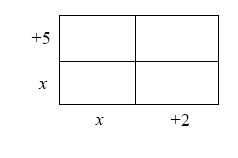### Home > GC > Chapter 3 > Lesson 3.2.6 > Problem3-100

3-100.

The area of the rectangle shown below is $40$ square units. Write and solve an equation to find $x$. Then find the dimensions of the rectangle.Complete the multiplication in the generic rectangle.
The sum of the small rectangles is $40$ square units.